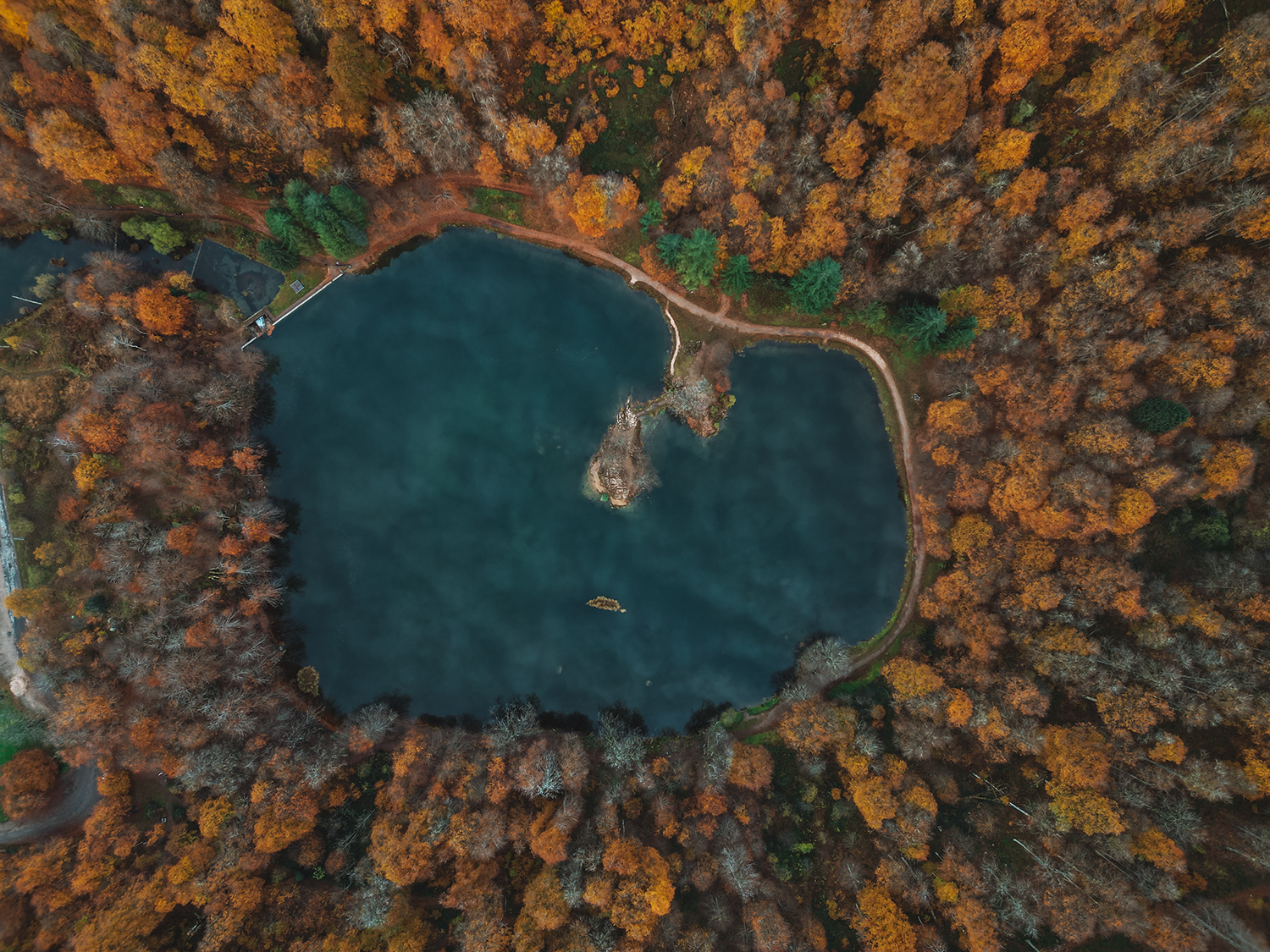## How to Calculate Pond Size and Volume

We are frequently asked for help in calculating the surface area and volume of ponds. Knowing these measurements is necessary information if you plan to add pond chemicals or dyes or even to properly aerate your water. Sometimes understanding the math to make the calculations can seem intimidating, but hopefully the following can help.

The most important part of any of these calculations is the underlying measurements. If the basic measurements are not accurate, the calculations will not provide good results. Use a tape measure rather than pacing it off if possible. You can also make length and width measurements using software available on the internet with considerable accuracy.

#### How to Find Surface Acreage

All length and width measurements are made in feet

For ponds that are basically rectangular:

1. Multiply the length of your pond by the width of your pond (this will give you the square footage).
2. Divide the square footage by 43,560. The result is your surface acreage.

For ponds that are basically round:

1. Multiply half of the pond width by half of the pond width.
2. Multiply your result by 3.142. The result is your square footage.
3. Divide your square footage by 43,560. The result is your surface acreage.

For large, irregular ponds and lakes, give us a call and we can use satellite imagery tools to measure your surface acreage.

#### How to Find the Average Depth of Your Pond

Depending on the size of your pond, you will probably need a small boat, canoe or kayak. Weight the end of a chain or rope. Take the rope out to the middle of your pond (wherever it is likely to be the deepest) and slowly let the weighted chain or rope down, until you feel the weight hit the bottom of the pond. Knot the rope or tie a string right above the water line to mark the water depth. When you get back to shore, measure the length from the weight to your waterline marker.

The average depth is usually approximately 2/3 of the depth at the deepest point, so simply take your measurement and divide by 3 then multiply the result by 2.

#### How to Find Acre-feet (Volume)

Multiply your Surface Acreage by the average depth of your pond. The result is a volume measurement known as acre-feet.

For example: If your pond is 2 surface acres, with an average depth of 10 feet, your pond volume is 20 acre-feet of water.

If you are a math major, an engineer, or astrophysicist, you have my apologies. I know that there are ways to write all of these things as specific equations, but for those of you that hated algebra class, these directions are written to help you think of these as just a sequential process. If you are still baffled: don’t worry, give us a call. We are always happy to help you with the math and any other pond questions you may have!